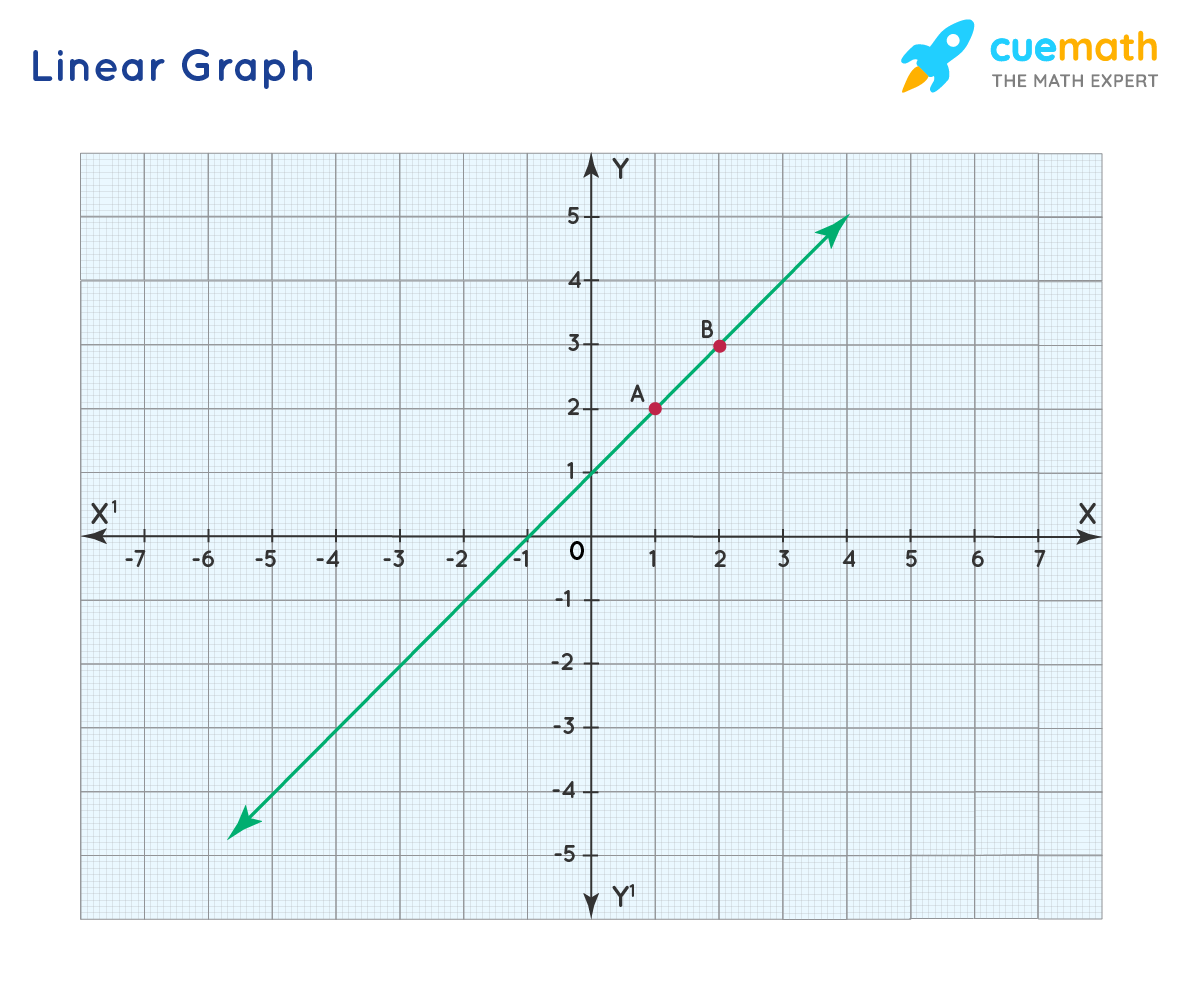# Linear Graph Calculator

## What Is a Linear Graph Calculator?

A linear graph is the graphical representation of a straight line which is represented by the equation of the form 'y = mx+c'. 'Cuemath's Online Linear Graph alculator' is a free online tool that sketches the linear graph passing through two points within a few seconds.

## How to Use the Linear Graph Calculator?

Follow the steps mentioned below to sketch the linear graph of the line passing through two points.

• Step 1- Enter the values of x1, x2, y1, y2.
• Step 2- Click on "Draw" to sketch the linear graph passing through those points.
• Step 3- Click on "Reset" to clear the fields and enter the new points.

## How to Draw a Linear Graph?

Consider the following steps to plot a linear graph:

• Select the two points (x1,y1), (x2, y2) to draw the linear graph.
• Mark the two points on the graph by using their x and y coordinates.
• Then use a ruler to join the two points with a line passing through those points.

The equation of the line can be found by substituting both the points in the linear equation y = mx+c. The slope 'm' and the vertical intercept 'c'  can be found using both the equations you get from the two points.

Let's learn through an example how to sketch a linear graph.

### Solved Example :

Sketch a linear graph passing through points A(1,2) and B(2,3).

### Solution :

In the first step, we have to mark the two points A and B on the graph paper. To mark point A, travel 1 unit towards the positive x-axis from the origin (0,0) then 2 units upward. To mark point B, travel 2 units towards the positive x-axis from the origin (0,0) then 3 units upward.

After marking the two points A and B use a ruler and sketch the line passing through the two points.Now, use linear graph calculator and sketch the graph of the following points.

1) (2,3), (3,4)

2) (5,6), (7,2)